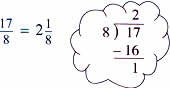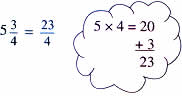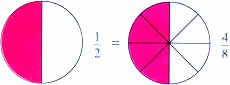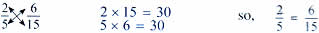Tuesday , May 26 2020# NCERT 5th Class (CBSE) Mathematics: Fractions

A faction shows a part of a whole.

A whole can be region or a collection.You are familiar with these fractions.

• Like Fractions: Fractions that have same denominator are called like fractions, e.g., 3/7, 5/7, 1/7
• Unlike fractions: Factions that have different denominators are called unlike fractions, e.g., 4/7, 3/8, 2/3
• Proper fractions: A fractions with the numerator less than the denominator is a proper fraction. A proper fraction has a value less than 1. For example, 1/8, 4/9, 3/5
• Improper fraction: A fraction that has the numerator greater that equal to the denominator is called an improper fraction and its value is 1 or more than 1. For example, 5/2, 10/7, 8/3, 9/9
• Mixed number: When we combine a whole number and a fraction, we get a mixed number. For example,Equivalent fractions: Fractions that name the same part are called equivalent fractions.You can find equivalent fractions by multiplying or dividing the numerator and the denominator by the same number.

1 (×4)/2 (×4) = 4/8

4 (÷2)/8 (÷2) = 2 (÷2)/4 (÷2) = 1/2

You can cross multiply to check whether two fractions are equivalent.## 10th Class CBSE Mathematics 2018-19

10th Class CBSE Mathematics 2018-19 Time: 3 hours M.M.: 80 Class: 10th Subject: Mathematics Date: 07/03/2019 …

### One comment

1.I need worksheet for Class 5th Mathematics.